# It is known that a force with a moment of 960 N · m about D is required to straighten the fence post CD. If the capacity of winch puller AB is 2400 N, determine the minimum value of distance d to create the specified moment about Point D.

Question-AnswerCategory: Engineering MechanicsIt is known that a force with a moment of 960 N · m about D is required to straighten the fence post CD. If the capacity of winch puller AB is 2400 N, determine the minimum value of distance d to create the specified moment about Point D.

It is known that a force with a moment of 960 N · m about D is required to straighten the fence post CD. If the capacity of winch puller AB is 2400 N, determine the minimum value of distance d to create the specified moment about Point D.Step: 1

Draw the free-body diagram of the fence post:Step: 2

From the geometry of the figure, state as follows:Here, the angle between ED and EC isand the distance between E and D is.

Step: 3

Consider the equilibrium of moments about point.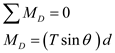Here, the moment acting at D isand the maximum tension in cable BC is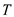.
Substituteand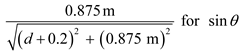in the above equation,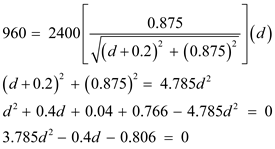Step: 4

Solve the above quadratic equation for the value of d.From the above data, the value of d should not be negative in sign. Hence, the value is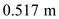.
Therefore, the minimum value of distance d to create a specified moment about the point D is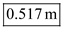.